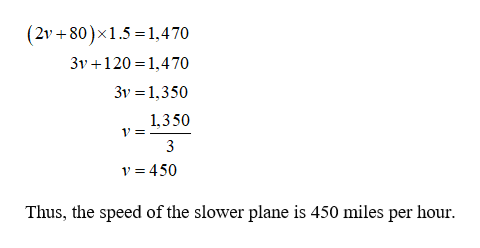# Two planes are 1470 mi apart and traveling toward each other. One plane is traveling 80 mph faster than the other plane. The planes pass each other in 1.5 h. Find the speed of each plane.slower plane    mphfaster planemph

Question
1 views

Two planes are 1470 mi apart and traveling toward each other. One plane is traveling 80 mph faster than the other plane. The planes pass each other in 1.5 h. Find the speed of each plane.

 slower plane mph faster plane mph

check_circle

Step 1

Let v be the velocity of the slower plane then the velocity of the faster plane is v + 80 and the planes pass each other in 1.5 hours.

That is, the two planes are 1,470 miles apart and one plane relative speed with respective to another is v + v + 80 = 2v +80.

It is known that, the product of time and speed is the value of travelled distance.help_outlineImage Transcriptionclose|(2v+80)x1.5 1,470 3v120 1.4 70 3v 1,350 1,350 1' = 3 v 450 Thus, the speed of the slower plane is 450 miles per hour fullscreen
Step 2

Substitute v = 450 in v + 80 to find the speed the faster plane as follows,

450+80 = 530.

Thus, the speed of the faster plane is 530 miles per hour.

Step 3

### Want to see the full answer?

See Solution

#### Want to see this answer and more?

Solutions are written by subject experts who are available 24/7. Questions are typically answered within 1 hour.*

See Solution
*Response times may vary by subject and question.
Tagged in

### Applications of Mathematics